# Tutorial 19: Unfitted Poisson

In this tutorial, we will learn

• How to use GridapEmbedded
• How to formulate an unfitted finite element method
• How to construct an unfitted boundary geometry setup
• How to use the Aggregated Finite Element Method
• How to impose Dirichlet boundary conditions weakly

## Problem statement

We want to solve the Poisson equation on the crescent moon 2D shape shown in the next figure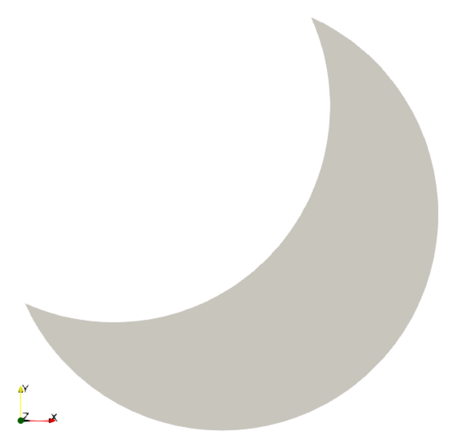We prescribe the linear solution to the problem $u(x) = x - y$ and Dirichlet boundary conditions on the whole boundary. Thus, the problem to solve is: find the scalar field $u$ such that

\left\lbrace \begin{aligned} -\Delta u &= 0 \ &\text{in} \ \Omega,\\ u &= x - y \ &\text{on}\ \partial\Omega \end{aligned} \right.

## Numerical scheme

To solve this PDE, we will use an unfitted technique. The idea behind unfitted, aka embedded or immersed boundary, methods is quite simple: Instead of relying on a mesh that fits to the domain boundary, we embed the domain in a background grid, upon which we will define our finite elements.

Body-fittedUnfitted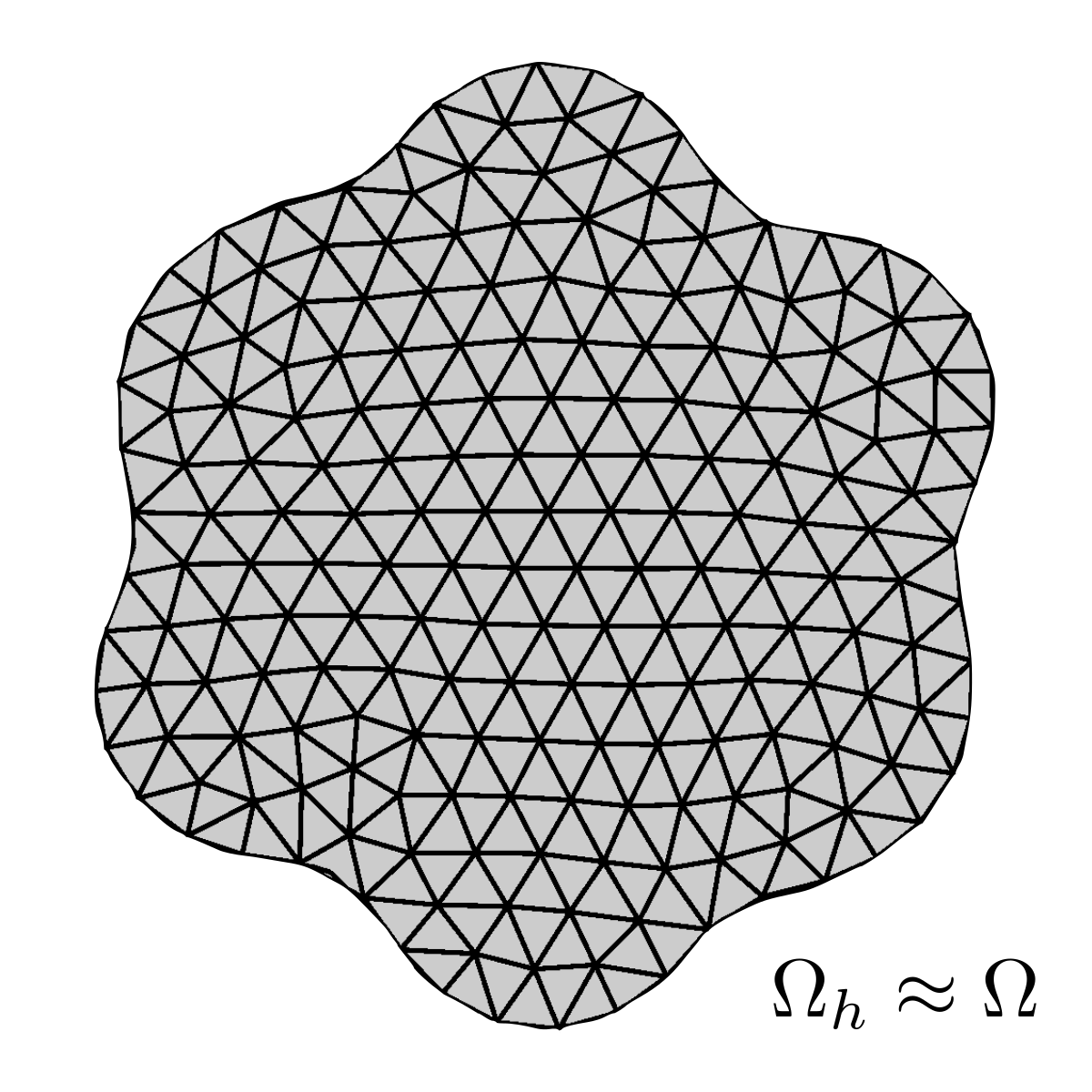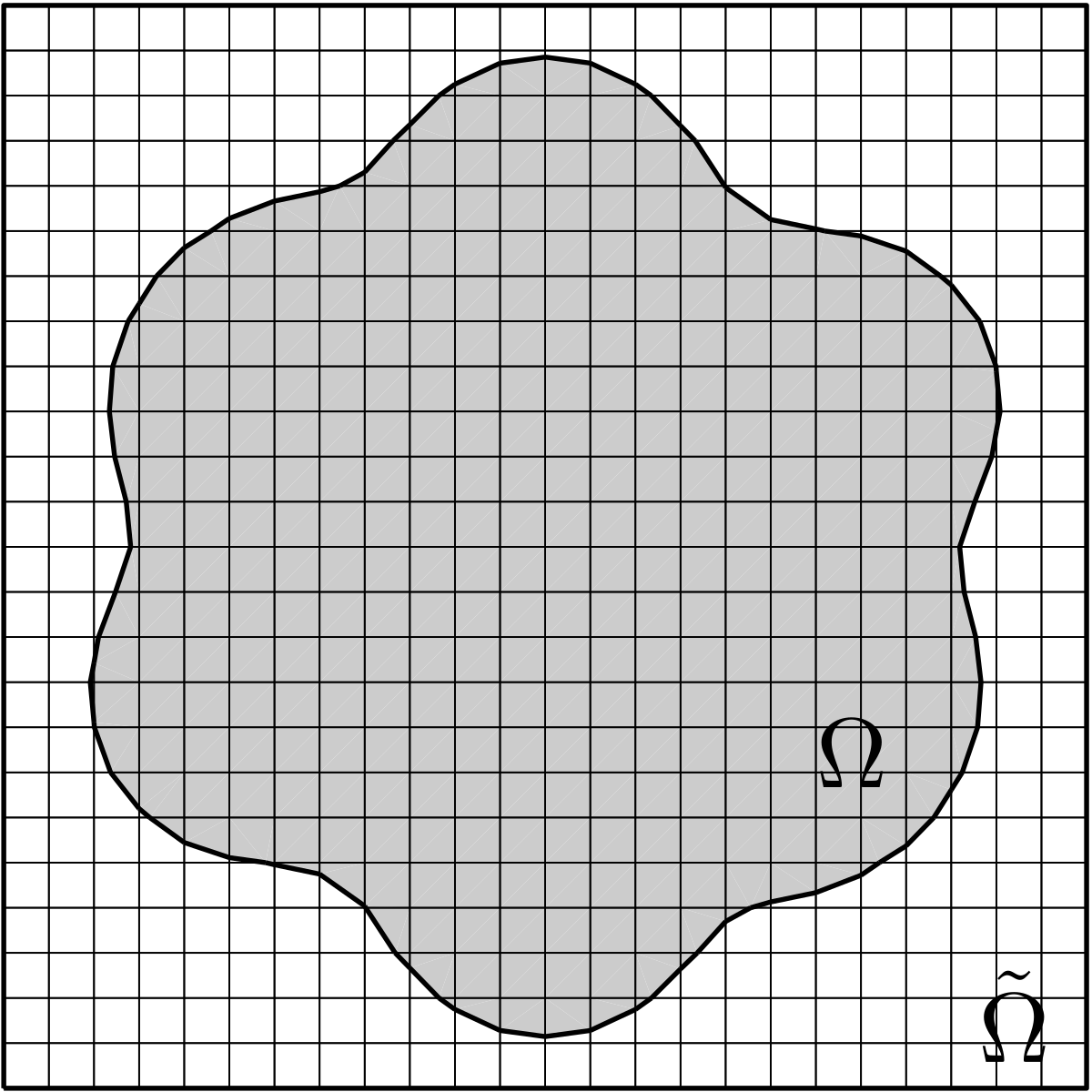Unfitted methods allow one to circumvent the meshing bottleneck when dealing with very complex geometries. But, as usual, this comes at a price. In unfitted methods, integration of the weak form and imposition of Dirichlet boundary conditions becomes more involved. In addition, we have to be careful with the small cut cell problem, which refers to stability and ill-conditioning issues that appear in presence of very small intersections between the background cells and the domain.

Fortunately, there are plenty of solutions available to take care of all these challenges. For more details, we refer to this recent review:

F. de Prenter, C. Verhoosel, H. van Brummelen, M. Larson, S. Badia, Stability and conditioning of immersed finite element methods: analysis and remedies arXiv preprint arXiv:2208.08538

In this tutorial, we will use the functionality available at GridapEmbedded to formulate and solve the unfitted problem above. We will illustrate a typical unfitted FE simulation pipeline in GridapEmbedded, including how we setup the embedded geometry, how we deal with the integration of the weak form and the imposition of Dirichlet boundary conditions.

We will discretise the problem with the aggregated finite element method, which is robust to the small cut cell problem. For more details, we refer to:

S. Badia, A.F. Martín, F. Verdugo, The aggregated unfitted finite element method for elliptic problems, Computer Methods in Applied Mechanics and Engineering 336 (2018), 533-553.

We recommend to read both references above to grasp the details of this tutorial.

The first step is to load the Gridap and GridapEmbedded packages.

using Gridap
using GridapEmbedded

### Implicit boundary representation

The next step is to generate the embedded geometry. The most straightforward way to represent the geometry is with the level-set method. It amounts to represent a closed curve (2D) or surface (3D) $\Gamma$ using an auxiliary function $\psi$, called the level-set function. $\Gamma$ is represented as the zero-level-set of $\psi$ by

$\Gamma = \{ (x,y) \ | \ \psi(x,y) = 0 \}$

and the level-set method manipulates $\Gamma$ implicitly, through the function $\psi$. This function $\psi$ is assumed to take negative values inside the region delimited by $\Gamma$ and positive values outside.

GridapEmbedded offers out-of-the-box level set surface descriptions for the disk, cylinder, sphere, square, tube, among others. You can also implement your own level-set function.

Interestingly, you can also construct complex domains from simple level-set descriptions. This is done by translating Boolean set operations and geometric transformations into simple manipulations of the level-set functions. For instance, given two level-set functions $\psi_1$ and $\psi_2$, representing the domains $\Omega_1$ and $\Omega_2$, the level-set function $\psi = \min(\psi_1,\psi_2)$ gives the domain $\Omega_1 \cup \Omega_2$.

We will use the former properties to generate the crescent moon shape with GridapEmbedded as follows. First, we note that the crescent moon shape is the outcome of subtracting to a disk of radius $R=1/2$ another disk of radius R, whose center is at a relative position $(-R/2,R/2)$.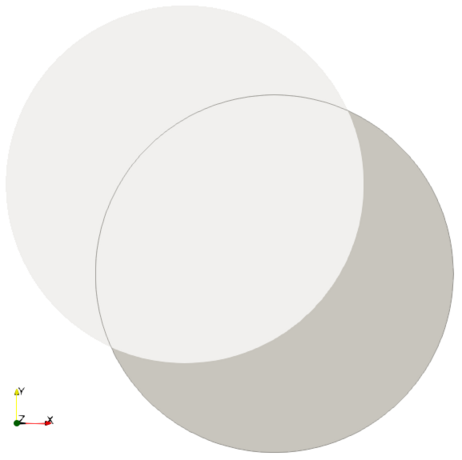Defining this geometry in GridapEmbedded is done as follows:

R  = 0.5
L  = 0.5*R
p1 = Point(0.0,0.0)
p2 = p1 + VectorValue(-L,L)

geo1 = disk(R,x0=p1)
geo2 = disk(R,x0=p2)
geo3 = setdiff(geo1,geo2)

Where we have used the setdiff function to subtract the disk geo2 to the disk geo1. This is a very simple example of constructive solid geometry. GridapEmbedded allows to create more complex CSG geometries as shown here. For more general shapes, GridapEmbedded admits an STL description of the implicit geometry, see package STLCutters for more details.

### Unfitted triangulations

As explained, the rationale of unfitted methods is to embed the domain of interest in a background Cartesian grid (or, more generally, any easy-to-generate mesh). In our case, we generate a 30x30 Cartesian grid model containing the geometry as

t = 1.01
pmin = p1-t*R
pmax = p1+t*R

n = 30
partition = (n,n)
bgmodel = CartesianDiscreteModel(pmin,pmax,partition)
dp = pmax - pmin

Unfitted FE formulations in GridapEmbedded hang on two different type of triangulations: "Active" and "Physical". In order to generate them, we need first to cut the embedded geometry against the model with the function cut.

cutgeo = cut(bgmodel,geo3)

cut generates an EmbeddedDiscretization instance (here, cutgeo). An EmbeddedDiscretization classifies all cells and facets from the background model into inside, outside or cutting the embedded geometry. Cells and facets of the background model outside the embedded geometry play no role in the unfitted formulation, they are inactive, thus it is convenient to remove them and restrict the triangulations to the active portion of the model (i.e., cut and interior cells and facets). In GridapEmbedded we can do this using the classification from cutgeo and the ACTIVE keyword.

Ω_act = Triangulation(cutgeo,ACTIVE)

To illustrate this concept, we can plot both the background and active triangulations to compare them.

Ω_bg = Triangulation(bgmodel)
writevtk(Ω_bg,"bg_trian")
writevtk(Ω_act,"act_trian")

In the picture below of the background grid, white cells are inactive, whereas gray cells are active.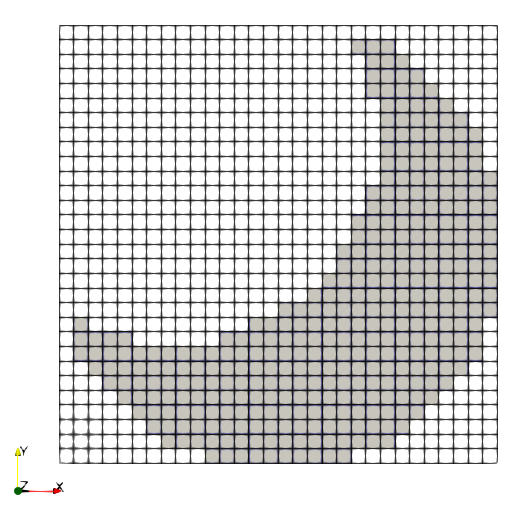As we will see later, we define our unfitted FE spaces on active triangulations.

An EmbeddedDiscretization instance (here, cutgeo) also generates subtriangulations on each cut cells to represent the portion of the cell which is inside the domain of analysis. We use these subtriangulations to generate the so called physical triangulations. Physical triangulations are nothing other than a body-fitted mesh of our domain $\Omega$, but we only use them to integrate the weak form of the problem in $\Omega$, we won't define FE spaces and assign DoFs on top of them. In GridapEmbedded we build physical triangulations using the PHYSICAL keyword.

Ω = Triangulation(cutgeo,PHYSICAL)
writevtk(Ω,"phys_trian")

Once again, we can combine plots of the physical and active triangulations to illustrate these concepts. In the first plot, we show the physical triangulation within the background one.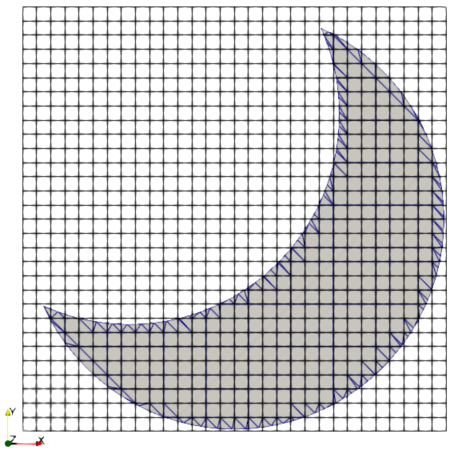Note that cut cells are subtriangulated to approximate the embedded geometry, as exposed in the following close-up plot.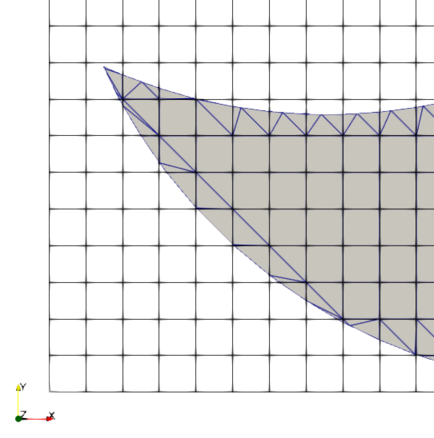In the third plot, we show the region represented by physical triangulation (shaded in gray) embedded in the active grid (black-contoured cells).In a nutshell, to define the unfitted FE formulation of the problem we need the \"active\" and \"physical\" triangulations of the domain. The former triangulation is used to define the FE spaces, whereas the latter is used to integrate the weak form. We use a level-set function to derive both of them.

ActivePhysical
For FE spacesFor measures### Unfitted FE spaces

Standard FE methods, i.e. the usual ones for body-fitted meshes, are exposed to the small cut cell problem, which leads to stability and conditioning issues due to the presence of arbitrarily small cut cells. In unfitted FEM, it is impractical to have control over how the mesh intersects the geometry, so we need to treat these numerical issues.

As said, in this tutorial, we leverage the aggregated unfitted finite element method (AgFEM), see the figure below. The main idea is to remove DoFs on cut cells, whose basis functions potentially have very small local support. In order to do that, we constrain exterior DoFs ${\color{red}\times}$ in terms of interior DOFs ${\color{blue}\bullet}$ (see figure below) as follows:

1. We map every exterior DoF ${\color{red}\times}$ to an interior cell $\tilde{K}({\color{red}\times})$ of the active triangulation using a cell aggregation scheme (thus the name of the method).
2. We extrapolate the value at the exterior DoF ${\color{red}\times}$ with the local FE basis at the interior cell $\tilde{K}({\color{red}\times})$. This leads to:
$u_{{\color{red}\times}} = \sum_{{\color{blue}\bullet}\in \tilde{K}({\color{red}\times})} \varphi_{{\color{blue}\bullet}}(x_{{\color{red}\times}})u_{{\color{blue}\bullet}}, \quad \forall {\color{red}\times}$

Hence, we end up extrapolating exterior DoFs ${\color{red}\times}$, in terms of interior ones ${\color{blue}\bullet}$.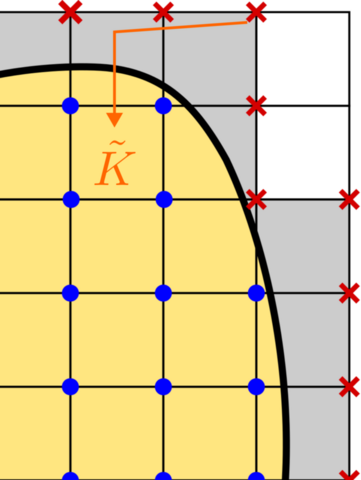We define the AgFEM space of our problem as follows. First, we generate a standard linear FE space on the ACTIVE triangulation. We do not prescribe Dirichlet boundaries on the standard FE space for reasons that will be clear later in the tutorial.

order = 1
reffe = ReferenceFE(lagrangian,Float64,order)
Vstd = TestFESpace(Ω_act,reffe,conformity=:H1)

After this, we construct the aggregates which will be used to build the map of exterior DoFs to interior ones.

strategy = AggregateAllCutCells()
aggregates = aggregate(strategy,cutgeo)

Note the following remark:

Here, we aggregate all cut cells. Alternatively, we can leave out large enough cut cells using AggregateCutCellsByThreshold(t), where t is a real number. Given a cut cell, if the ratio of the cut region volume and the background cell volume is larger than t, then this cell is not aggregated.

We can color the aggregates and easily inspect them on the background mesh.

colors = color_aggregates(aggregates,bgmodel)
Ω_bg = Triangulation(bgmodel)
writevtk(Ω_bg,"aggs_on_bg_trian",celldata=["aggregate"=>aggregates,"color"=>colors])

Finally, we use the aggregates to constrain the exterior DoFs of Vstd in terms of the interior ones. This leads to the AgFEM space.

V = AgFEMSpace(Vstd,aggregates)
U = TrialFESpace(V)

Note that V is a test FE space and the trial FE space U does not receive Dirichlet functions, because we have not prescribed Dirichlet boundaries in the standard test FE space Vstd.

### Unfitted measures

We define next the integration measures on the PHYSICAL triangulation.

degree = 2*order
dΩ = Measure(Ω,degree)

Although degree = 2*order is enough for this particular linear case. In general, we need to enforce degree = 2*dim*order on cut cells. This is a detail. We just leave a brief explanation of the reason below.

Since interior cells are rectangular, Fubini theorem applies and we can separate the integrals of the weak form by dimensions. Thus, we can take as quadrature degree 2*order as usual. However, in the case of cut cells, Fubini theorem does not hold. So, to take into account that we cannot separate the integrals, the quadrature degree on cut cells must be premultiplied by the problem dimension, i.e. degree = 2*dim*order.

### Imposing Dirichlet boundary conditions

In unfitted FEMs, we cannot impose Dirichlet boundary contions on the Poisson problem as in body-fitted ones. The reason is apparent in the figure below.

We assume a Poisson problem defined on a 2D shape with Dirichlet $\Gamma_D$ and Neumann $\Gamma_N$ BCs and a close-up on the Dirichlet boundary.

Model problemBody-fittedUnfitted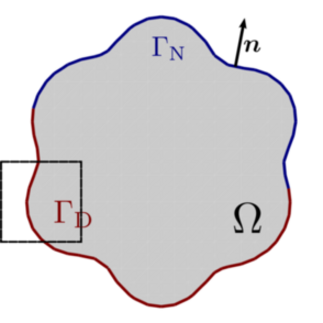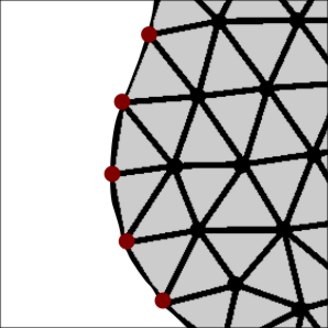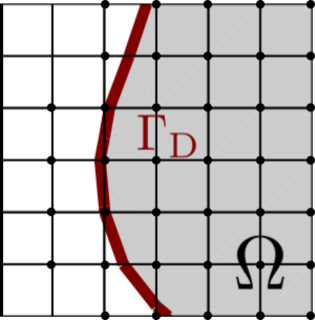Clearly, on body-fitted meshes, we can impose Dirichlet BCs strongly, i.e. remove the basis functions associated to Dirichlet DoFs from the FE space. On unfitted meshes, we must enforce these conditions weakly. In order to do that we resort to Nitsche's method:

\begin{aligned} a_K(u,v) &\doteq \int_{\Omega \cap K} \nabla u \cdot \nabla v \ \mathrm{d}\Omega + \int_{\Gamma_{\rm D} \cap K} \beta_K uv - (\nabla u \cdot \boldsymbol{n} ) v - (\nabla v \cdot \boldsymbol{n} ) u \ \mathrm{d}\Omega \\ l_K(v) &\doteq \int_{\Omega \cap K} f v \ \mathrm{d}\Omega + \int_{\Gamma_{\rm N} \cap K} g v \ \mathrm{d}\Gamma + \int_{\Gamma_{\rm D} \cap K} \beta_K u_{\rm D}v - (\nabla v \cdot \boldsymbol{n} ) u_{\rm D} \ \mathrm{d}\Omega \end{aligned}

where $\beta_K$ must be large enough to ensure coercivity of the problem.

This means we need to integrate boundary tems along the embedded boundary. For this purpose we generate the embedded boundary triangulation and measure.

Γ = EmbeddedBoundary(cutgeo)
n_Γ = get_normal_vector(Γ)
dΓ = Measure(Γ,degree)

We can now write the weak form of the problem.

u(x) = x - x # Solution of the problem
const γd = 10.0    # Nitsche coefficient
const h = dp/n  # Mesh size according to the parameters of the background grid

a(u,v) =
∫( ∇(v)⋅∇(u) )dΩ +
∫( (γd/h)*v*u  - v*(n_Γ⋅∇(u)) - (n_Γ⋅∇(v))*u )dΓ

l(v) = ∫( (γd/h)*v*u - (n_Γ⋅∇(v))*u )dΓ

Once we have done all this, the rest of the pipeline follows the steps of a standard FE simulation.

op = AffineFEOperator(a,l,U,V)
uh = solve(op)

e = u - uh

l2(u) = sqrt(sum( ∫( u*u )*dΩ ))
h1(u) = sqrt(sum( ∫( u*u + ∇(u)⋅∇(u) )*dΩ ))

el2 = l2(e)
eh1 = h1(e)
ul2 = l2(uh)
uh1 = h1(uh)

using Test
@test el2/ul2 < 1.e-8
@test eh1/uh1 < 1.e-7

writevtk(Ω,"results.vtu",cellfields=["uh"=>uh])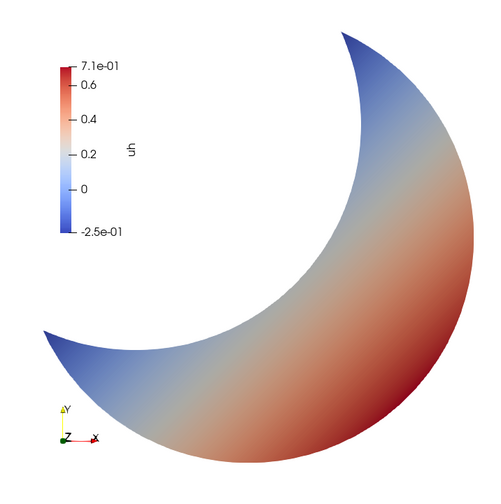Tutorial done!